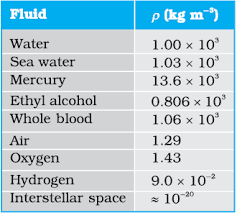# Pressure

A sharp needle when pressed against our skin pierces it. Our skin, however, remains intact when a blunt object with a wider contact area (say the back of a spoon) is pressed against it with the same force. If an elephant were to step on a man’s chest, his ribs would crack. A circus performer across whose chest a large, light but strong wooden plank is placed first, is saved from this accident. Such everyday experiences convince us that both the force and its coverage area are important. Smaller the area on which the force acts, greater is the impact. This impact is known as pressure.

Fig. 1 (a) The force exerted by the liquid in the beaker on the submerged object or on the walls is normal (perpendicular) to the surface at all points. (b) An idealised device for measuring pressure.

When an object is submerged in a fluid at rest, the fluid exerts a force on its surface. This force is always normal to the object’s surface. This is so because if there were a component of force parallel to the surface, the object will also exert a force on the fluid parallel to it; as a consequence of Newton’s third law. This force will cause the fluid to flow parallel to the surface. Since the fluid is at rest, this cannot happen. Hence, the force exerted by the fluid at rest has to be perpendicular to the surface in contact with it. This is shown in Fig.1(a).

The normal force exerted by the fluid at a point may be measured. An idealised form of one such pressure-measuring device is shown in Fig. 1(b). It consists of an evacuated chamber with a spring that is calibrated to measure the force acting on the piston. This device is placed at a point inside the fluid. The inward force exerted by the fluid on the piston is balanced by the outward spring force and is thereby measured.

If F is the magnitude of this normal force on the piston of area A then the average pressure $P_{av}$ is defined as the normal force acting per unit area.

$P_{av}=\frac{F}{A}$

In principle, the piston area can be made arbitrarily small. The pressure is then defined in a limiting sense as

$P_{av}=\lim_{\Delta A\rightarrow 0 } \frac{\Delta F}{\Delta A}$

Pressure is a scalar quantity. We remind the reader that it is the component of the force normal to the area under consideration and not the (vector) force that appears in the numerator in Eqs. (10.1) and (10.2). Its dimensions are [$ML^{-1}T^{-2}$]. The SI unit of pressure is N.m$^{–2}$. It has been named as pascal (Pa) in honour of the French scientist Blaise Pascal (1623-1662) who carried out pioneering studies on fluid pressure. A common unit of pressure is the atmosphere (atm), i.e. the pressure exerted by the atmosphere at sea level (1 atm = 1.013 × 10$^5$  Pa).

Another quantity, that is indispensable in describing fluids, is the density ρ. For a fluid of mass m occupying volume V,

$\rho = \frac{m}{V}$

The dimensions of density are [ML$^{–3}$]. Its SI unit is kg.m$^{–3}$. It is a positive scalar quantity. A liquid is largely incompressible and its density is therefore, nearly constant at all pressures. Gases, on the other hand exhibit a large variation in densities with pressure.

The density of water at 4$^o$C (277 K) is 1.0 × 10$^3$ kg.m$^{–3}$. The relative density of a substance is the ratio of its density to the density of water at 4$^o$C. It is a dimensionless positive scalar quantity. For example the relative density of aluminium is 2.7. Its density is 2.7 × 10$^3$  kg.m$^{–3}$ . The densities of some common fluids are displayed in Table 1.Table 1 Densities of some common fluids at STP*

Example 1
The two thigh bones (femurs), each of cross-sectional area10 cm$^2$ support the upper part of a human body of mass 40 kg. Estimate the average pressure sustained by the femurs.

Total cross-sectional area of the femurs is A = 2 × 10 cm$^2$  = 20 × 10$^{–4} m$^2$. The force acting on them is F = 40 kg wt = 400 N (taking g = 10 m.s$^{–2}$). This force is acting vertically down and hence, normally on the femurs. Thus, the average pressure is$P_{av}=\frac{F}{A}=2 \times 10^5\$ Pa